#### Thank you for registering.

One of our academic counsellors will contact you within 1 working day.

Click to Chat

1800-5470-145

+91 7353221155

CART 0

• 0
MY CART (5)

Use Coupon: CART20 and get 20% off on all online Study Material

ITEM
DETAILS
MRP
DISCOUNT
FINAL PRICE
Total Price: Rs.

There are no items in this cart.
Continue Shopping

# Chapter 5: Trigonometric Ratios Exercise – 5.2

### Question: 1

sin 45° sin 30° + cos 45° cos 30°

### Solution:

Sin 45°sin 30° + cos 45° cos 30°      

We know that by trigonometric ratios we have,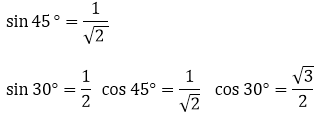Substituting the values in equation 1, we get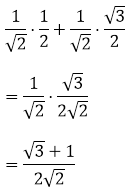### Question: 2

sin 60° cos 30° + cos 60° sin 30°

### Solution:

sin 60° cos 30° + cos 60° sin 30° 

By trigonometric ratios we have,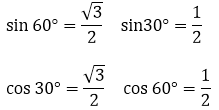Substituting the values in equation 1, we get### Question: 3

cos 60° cos 45° – sin 60° sin 45°

### Solution:

cos 60° cos 45° – sin 60° sin 45°  

We know that by trigonometric ratios we have,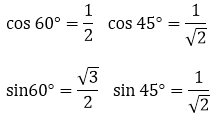Substituting the values in equation 1, we get### Question: 4

sin230° + sin245° + sin260° + sin290°

### Solution:

sin230° + sin245° + sin260° + sin290°   

We know that by trigonometric ratios we have,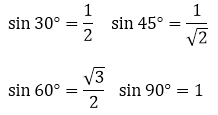Substituting the values in equation 1, we get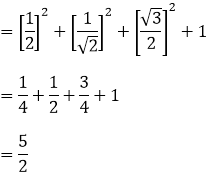### Question: 5

cos230° + cos245° + cos260° + cos290°

### Solution:

cos230° + cos245° + cos260° + cos290° 

We know that by trigonometric ratios we have,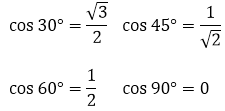Substituting the values in equation 1, we get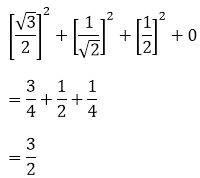### Question: 6

tan230° + tan245° + tan260°

### Solution:

tan230° + tan245° + tan260°          

We know that by trigonometric ratios we have,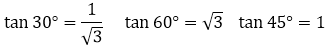Substituting the values in equation 1, we get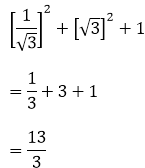### Question: 7

2 sin230° − 3 cos245° + tan260°

### Solution:

2sin230° − 3cos245° + tan260°         

We know that by trigonometric ratios we have,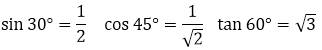Substituting the values in equation 1, we get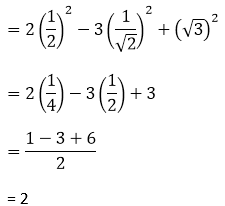### Question: 8

sin2 30° cos245°+ 4 tan230°+ 1/2sin2 90° - 2 cos2 90°+ 1/24 cos2

### Solution:

sin2 30° cos245°+ 4 tan230°+ 1/2sin2 90° - 2 cos2 90°+ 1/24 cos20° 

We know that by trigonometric ratios we have,Substituting the values in equation 1, we get### Question: 9

4(sin460° + cos430°) − 3(tan260° − tan245°) + 5cos245°

### Solution:

4(sin460° + cos430°) − 3(tan260° − tan245°) + 5cos245°   

We know that by trigonometric ratios we have,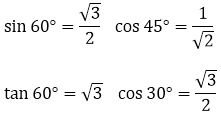Substituting the values in equation 1, we getQuestion: 10

(cosec2 45° sec2 30°) (sin230° + 4 cot245° − sec2 60°)

### Solution:

(cosec245° sec230°)(sin230° + 4 cot245° − sec260°)      

We know that by trigonometric ratios we have,Substituting the values in equation 1, we get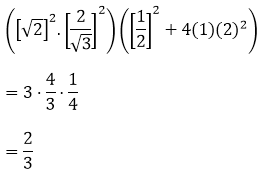### Question: 11

cosec3 30°cos 60° tan3 45° sin2 90° sec2 45°cot 30°

### Solution:

= cosec3 30°cos 60° tan3 45° sin2 90° sec2 45° cot 30°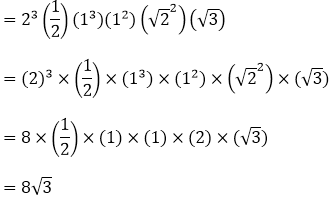### Question: 12

cot230° − 2cos260° − 3/4 sec245° – 4 sec230°

### Solution:

= cot230° − 2cos260° − 3/4 sec245° – 4 sec230°### Question: 13

(cos 0° + sin 45° + sin 30°) (sin 90 − cos 45° + cos 60°)

### Solution:

Given, (cos 0° + sin 45° + sin 30°) (sin 90 − cos 45° + cos 60°)### Question: 14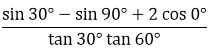### Solution:

Given,### Question: 15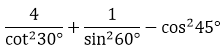### Solution:

Given,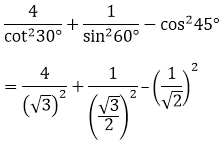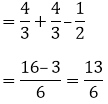### Question: 16

4(sin430° + cos260°) − 3(cos245° − sin290°) − sin260°

### Solution:

Given,

4(sin430° + cos260°) − 3(cos245° − sin290°) − sin260°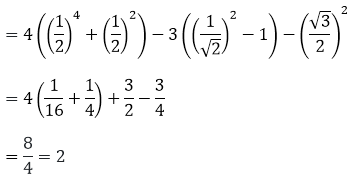### Question: 17### Solution:

Given,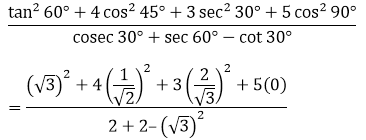= 3 + 2 + 4

= 9

### Question: 18### Solution:

Given,### Question: 19### Solution: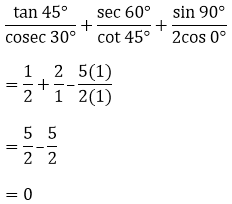2 sin 3x = √3

### Solution:

Given,

2 sin 3x = √3

⟹ sin 3x = √3/2

⟹ sin 3x = sin 60°

⟹ 3x = 60°

⟹ x = 20°

### Question: 21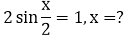### Solution: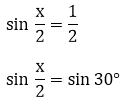x/2 = 30°

x = 60°

√3 sin x = cos x

### Solution:

√3 tan x = 1

tan x = 1/√3

∴ tan x =  tan45°

x = 45°

### Question: 23

tan x = sin 45° cos 45° + sin 30°

### Solution: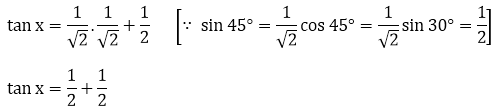tan x = 1

tan x = 45°

x = 45°

### Question: 24

√3 tan 2x = cos 60°+ sin 45°cos 45°

### Solution: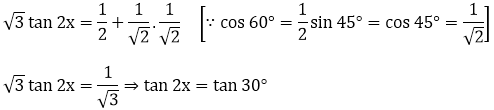2x = 30°

x = 15°

### Question: 25

cos 2x = cos 60° cos 30° + sin 60° sin 30°

### Solution: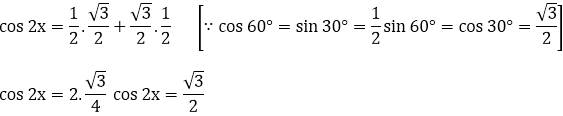2x = 30°

x = 15°

### Question: 26

If θ = 30°, verify(iv) cos 3θ = 4cos3θ − 3cos θ cos 3θ = 4 cos3 θ 3 cos θ

### Solution:Substitute θ = 30° θ = 30° in equation (i)Therefore, LHS = RHS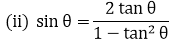Substitute θ = 30° θ = 30°Therefore, LHS = RHS.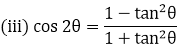Substitute θ = 30°θ = 30°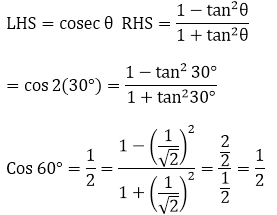Therefore, LHS = RHS

(iv) cos 3θ = 4cos3θ − 3cos θ cos 3θ = 4 cos3θ – 3 cos θ

Solution:

LHS = cos 3θ

Substitute θ = 30°

= cos 3(30°) = cos 90°

= 0

RHS = 4 cos3θ – 3 cos θ

= 4 cos330° − 3 cos 30°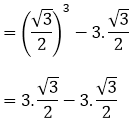= 0

Therefore, LHS = RHS.

### Question: 27

If A = B = 60°. Verify

(i) cos (A – B) = cos A cos B + sin A sin B

### Solution:

cos (A – B) = cos A cos B + sin A sin B … (i)

Substitute A and B in (i)

⟹ cos (60° – 60°) = cos 60° cos 60° + sin 60° sin 60°⟹ 1 = 1

Therefore, LHS = RHS

(ii) Substitute A and B in (i)

⟹ sin (60° – 60°) = sin 60° cos 60° – cos 60° sin 60°

⟹ sin0° = 0

⟹ 0 = 0

Therefore, LHS = RHSA = 60°, B = 60° we get,tan 0° = 0

0 = 0

Therefore, LHS = RHS

### Question: 28

If A = 30°, B = 60° verify:

(i) Sin (A + B) = Sin A cos B + Cos A Sin B

(ii) Cos (A + B) = Cos A Cos B – Sin A Sin B

### Solution:

(i) A = 30°, B = 60° we get

Sin (30° + 60°) = Sin 30° Cos 60° + Cos 30°  Sin 60°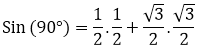Sin (90°) = 1 ⟹ 1 = 1

Therefore, LHS = RHS

(ii) Cos (A + B) = Cos A Cos B – Sin A Sin B

A = 30°, B = 60° we get

Cos (30° + 60°) = Cos 30° Cos 60° – Sin 30° Sin 60°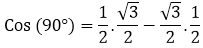0 = 0

Therefore, LHS = RHS

### Question: 29

If sin(A + B) = 1 and cos(A - B) = 1, 0° < A + B ≤ 90°, A ≥ B find A and B.

### Solution:

Given,

sin(A + B) = 1 this can be written as sin (A + B) = sin (90°) sin (90°)

cos(A - B) = 1 this can be written as cos (A - B) = cos (0°) cos (0°)

⟹ A + B = 90°

A – B = 0°

2A = 90°

A = 90°/2

A = 45°

Substitute A value in A – B = 0°

45°– B = 0°

B = 45°

Hence, the value of A = 45°and B = 45°

### Question: 30

If tan (A - B) = 1/√3 and tan (A + B) = √3, 0°<A + B ≤ 90°, A > B find A and B

### Solution:

Given,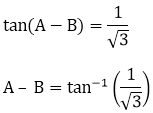A – B = 30°   ... 1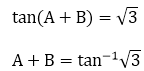A + B = 60° ... 2

Solve equations 1 and 2

A + B = 30°

A – B = 60°

2A = 90°

A = 90°/2

A = 45°

Substitute the value of A in equation 1

45°+ B = 30°

B = 30° – 45°

B = 15°

The value of A = 45°and B = 15°

### Question: 31

If sin (A - B) = 1/2 and cos (A + B) = 1/2, 0°<A + B ≤ 90°, A < B. find A and B.

### Solution:

Given,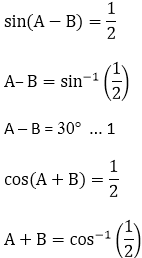A + B = 60° ... 2

Solve equations 1 and 2

A + B = 60°

A – B = 30°

2A  = 90°

A = 90°/2

A = 45°

Substitute the value of A in equation 2

45°+ B = 60°

B = 60° – 45°

B = 15°

The value of A = 45°and B = 15°

### Question: 32

In a Δ ABC right angled triangle at B, ∠A = ∠C. Find the values of:

1. sin A cos C + cos A sin C

2. sin A sin B + cos A cos B

### Solution:

1. since, it is given as ∠A = ∠C

the value of A and C is 45°, the value of angle  B is 90°

because the sum of angles of triangle is 180°

⟹ sin 45° cos 45° + cos 45° sin 45°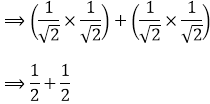⟹ 1

The value of sin A cos C + cos A sin C is 1

2. since, it is given as  ∠A = ∠C

the value of A and C is 45°, the value of angle  B is 90°

because the sum of angles of triangle is 180°

⟹ sin 45°sin 90° + cos 45°sin 90°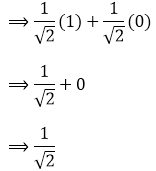The value of sin A sin B + cos A cos B is 1/√2

### Question: 33

Find the acute angle A and B, if sin (A + 2B) = √3/2 and cos(A + 4B) = 0, A > B.

### Solution:

Given,A + 2B = 60° ... 1

Cos (A + 4B) = 0

A + 4B = sin−1(90) sin−1(90)

A + 4B = 90°    ... 2

Solve equations 1 and 2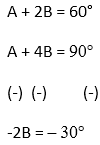2B = 30°

B = 30°/2

B = 15°

Substitute B value in equation 2

A + 4B = 90°

A + 4(15°) = 90°

A + 60° = 90°

A = 90° – 60°

A = 30°

The value of A = 30° and B = 15°

### Question: 34

In ΔPQR, right angled at Q, PQ = 3 cm and PR = 6 cm. Determine ∠ P and ∠ R.

### Solution:

In ΔPQR, right angled at Q, PQ = 3 cm and PR = 6 cm

By Pythagoras theorem,

PR2 = PQ2 + QR2

⟹ 62 = 32 + QR2

⟹ QR2 = 36 – 9

⟹ QR = √27

⟹ QR = 3√3

sin R = 3/6 = 1/2 = sin30°

∠R = 30°

As we know, Sum of angles in a triangle = 180

∠P + ∠Q + ∠R = 180°

⟹ ∠P + 90° + 30° = 180°

⟹ ∠P = 180° – 120°

⟹ ∠P = 60°

Therefore, ∠R = 30°

And, ∠P = 60°

### Question: 35

If sin (A – B) = sin A cos B – cos A sin B and cos (A – B) = cos A cos B + sin A sin B, find the values of sin 15 and cos 15.

### Solution:

Given,

sin (A – B) = sin A cos B – cos A sin B

And, cos (A – B) = cos A cos B + sin A sin B

We need to find, sin 15 and cos 15.

Let A = 45 and B = 30

sin 15 = sin (45- 30) = sin 45 cos 30 – cos 45 sin 30cos 15 = cos (45- 30) = cos 45 cos 30 – sin 45 sin 30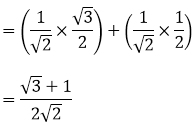### Question: 36

In a right triangle ABC, right angled at C, if ∠B = 60° and AB = 15 units. Find the remaining angles and sides.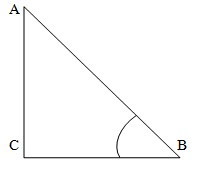### Solution:

Sin 60°= x/15cos 60°= x/15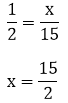x = 7.5 units

### Question: 37

In ΔABC is a right triangle such that ∠C = 90°, ∠A = 45°and BC = 7 units. Find the remaining angles and sides.

### Solution: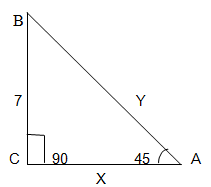Here, ∠C = 90° and ∠A = 45°

We know that,

∠A + ∠B + ∠C = 180°

⟹ 45°+ 90° + ∠C = 180°

⟹ 135° + ∠C = 180°

⟹ ∠C = 180° – 135°

⟹ ∠C = 45°

The value of the remaining angle C is 45°

Now, we need to find the sides x and y here,y =7√2 unitsx = 7 units

the value of x = 7 units and y = √2 units

### Question: 38

In a rectangle ABCD, AB = 20 cm, ∠BAC = 60°, calculate side BC and diagonals AC and BD.

### Solution:

Let AC = x cm and CB = y cm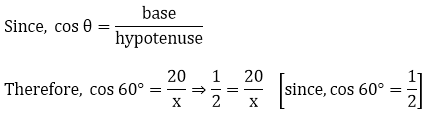⇒ x = 40 cm = AC

Similarly BD = 40 cm

Now,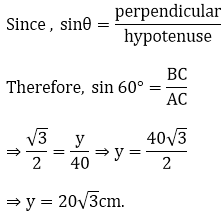### Question: 39

If A & B are acute angles such that tan A = 1/2 tan B = 1/3 and tan (A + B) =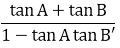find A+B.

### Solution: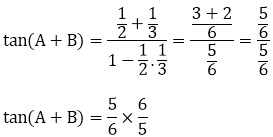(A + B) = Tan-1 (1)

(A + B) = 45°

### Question: 40

Prove that: (√3 -1) (3 – cot 30°) = tan360° - 2 sin 60°

### Solution:

R.H.S ⟹ tan60° - 2 sin 60°

L.H.S = R.H.S

Hence prove